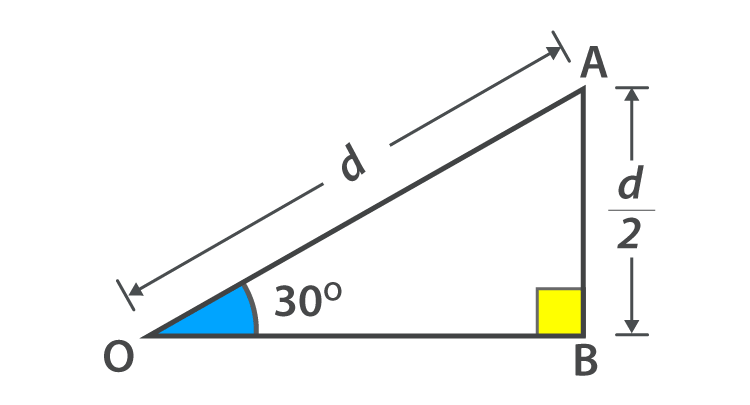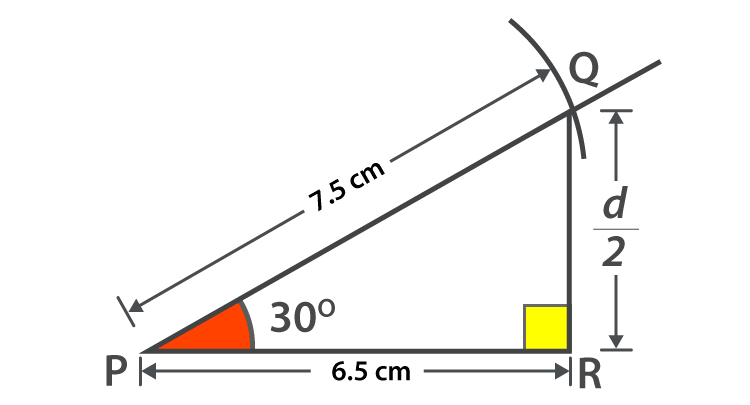# Cos 30 Degrees

In trigonometry, the cosine function is defined as the ratio of the adjacent side to the hypotenuse. If the angle of a right triangle is equal to 30  degrees, and then the value of cosine at this angle i.e., the value of Cos 30 degree is in a fraction form as √3/2. It is noted that the value of Sec 30 will be reciprocal of Cos 30 value.

## Cos 30 Value

Cos 30 degrees is written as cos 30° and has a value in fraction form as √3/2.

Cos 30° = √3/2

Cos 30° = √3/2 is an irrational number and equals to 0.8660254037 (decimal form).

Therefore, the exact value of cos 30 degrees is written as 0.8660 approx.

√3/2 is the value of Cos 30° which is a trigonometric ratio or trigonometric function of a particular angle.

## Cos 30

Another alternative form of Cos 30° is pi/6 or π/6 or Cos 33 (⅓)g

 Form Formula Value Trigonometric ratio √3 / 2 0.8660254037 Circular system pi/6 or π/6 0.8660254037 Centesimal system Cos 33 (⅓)g 0.8660254037

### Cos 30 Degrees Proof

Now that you know the value of Cos 30 degrees, let’s explore how to derive this value.

We will study the two approaches to derive it.

1. Theoretical approach
2. Practical approach

Theoretical approach

Knowing the property that length of an opposite side is half of the length of the hypotenuse – Right triangle property for an angle equal to 30 degrees in the given right triangle.

As we know two sides those are the length of the opposite side and hypotenuse but the third side is unknown i.e adjacent side. We need to find this side to find the value of Cos 30 degrees.We will use the Pythagorean theorem to find the value of this third side

In triangle AOB,

AO 2 = AB2 + AB2

Hypotenuse = d

Opposite side = d/2

d2 = (d/2)2 + AB2

d2 = d2/4 + AB2

d2 – d2/4 = AB2

(4d2 – d2)/4 = AB2

3d2/4 = AB2

Sqrt (3) x d/2 = AB

AB/d = √(3) / 2

As d is the length of the opposite side, so

Length of adjacent / length of hypotenuse = √3 / 2

The value of angle BOA in the given right triangle is pi/6 and the given ratio as per the definition of cosine ratio represents the value of Cos 30 degrees

Cos 30° =Length of adjacent/length of hypotenuse = √3/2 = 0.8660254037

Practical Approach

Another way to calculate the value of Cos 30 degree is through a practical approach. So if we make a right triangle using constructions, Cos pi/6 can be calculated.Step 1: Mark a point P on the plane and horizontally draw a line on it.

Step 2: With the help of a protractor makes an angle of 300 with the centre as P and taking the baseline as the drawn line in step 1.

Step 3: Use the ruler to draw a line to make an angle of 30 degrees.

Step 4: Using a compass, draw an arc on the line of an angle 30 degrees with any length. Mark that point as Q.

Step 5: From Q, draw a line perpendicular to the base (horizontal line). Mark it as R (Intersecting point of the perpendicular line to the base.)

Now after drawing a right-angled triangle PRQ, we can calculate the value of Cos 30 degrees.

Cos 30° =Length of the adjacent/length of the hypotenuse = PR/PQ.

In the above construction, the length of the side PQ is taken as 7.5 cm, and the length of the adjacent side is unknown. But if we measure it with a ruler, It comes out to be 6.5 cm.

Cos 30° = PR/PQ = 6.5/7.5 = 0.866666…

Stay tuned to BYJU’S to learn math in the most fun and engaging way.

 Related Links Cos 60 Degree Cos 0 Degree Cosine Function Cosine Rule
Quiz on Cos 30 Degrees